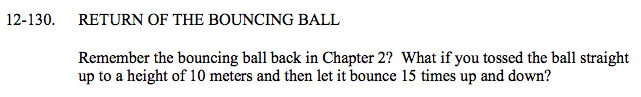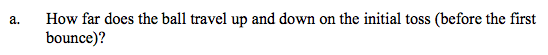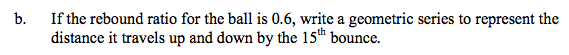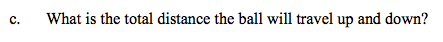### Home > A2C > Chapter 12 > Lesson 12.3.1 > Problem12-130

12-130.
1. RETURN OF THE BOUNCING BALL

2. Remember the bouncing ball back in Chapter 2? What if you tossed the ball straight up to a height of 10 meters and then let it bounce 15 times up and down? Homework Help ✎

1. How far does the ball travel up and down on the initial toss (before the first bounce)?

2. If the rebound ratio for the ball is 0.6, write a geometric series to represent the distance it travels up and down by the 15th bounce.

3. What is the total distance the ball will travel up and down?20 metersWhat is the initial value? What is the multiplier?

2(10 + 10(0.6) + ...?)49.976 meters